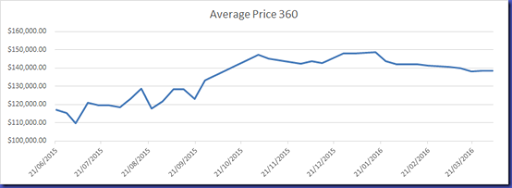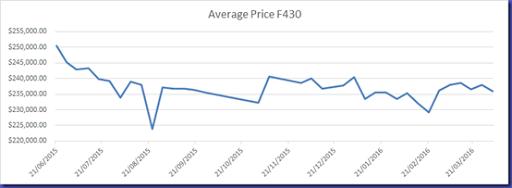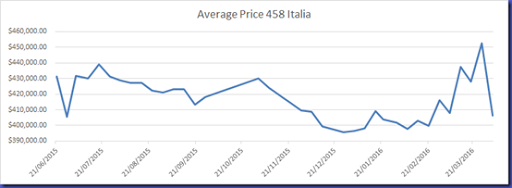# Weekly Price Guide–3 April 2016

F355 (All models)

Average = \$174,338.00

Change = \$3,665.50

360 (All models)

Average = \$138,739.10

Change = \$138.50F430 (All models)

Average = \$235,921.90

Change = -\$2,108.70458 Italia

Average = \$406,494.20

Change = -\$46,280.80458 Spider

Average = \$499,941.40

Change = \$0

Value = (Avg price / cars)

458 Spider = \$ 55,549.04

458 Italia = \$ 50,811.78

F355 = \$ 24,905.43

F430 = \$ 13,106.77

360 = \$ 6,032.13